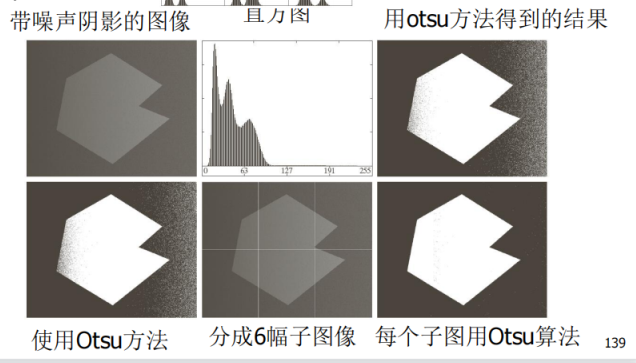# 数字图像处理复习笔记

[TOC]

## 第一章 绪论

### 数字图像的来源

1. 伽马射线成像：用于核医学和天文观测
2. X射线成像：用于医学、工业、天文
3. 紫外波段成像：荧光显微法
4. 可见光成像：最常见的
5. 红外波段成像：用于海岸线测绘、矿物测绘、土壤温度测绘、土壤含水量测绘
6. 微波波段成像：雷达图像，可以不被云层阻挡
7. 无线电波段成像：核磁共振成像MRI
8. 声波成像：医学超声波
9. 计算机生成图像

### 数字图像的特点是什么？

1. 信息量大
2. 像素之间相关性较大、冗余较多
3. 对三维景物的理解，使用单个视角二维图像不够
4. 二维图像的观察受人的主观因素较大

### 数字图像处理是什么？

• 狭义上指图像->图像，即通过处理把一副图像变为修改后的另一幅图像。
• 广义上也指图像->非图像，即理解分析一副数字图像。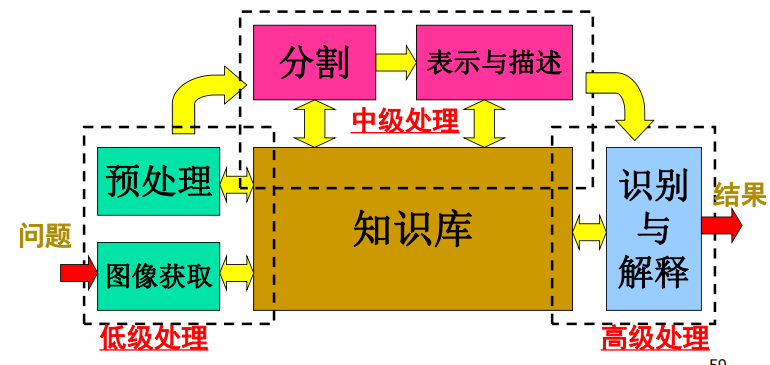## 第二章 数字图像基础

### 人类视觉感知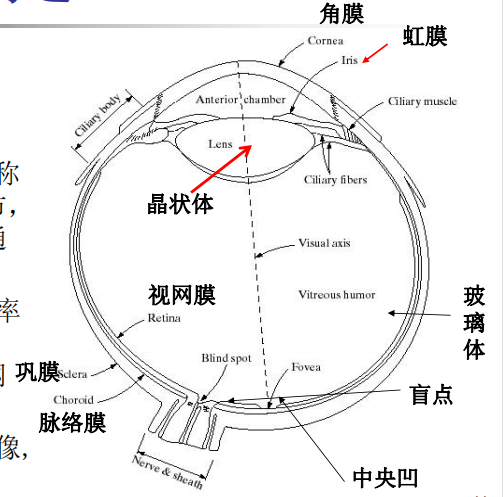• 虹膜：类似光圈，控制进光亮

• 晶状体：改变焦距、聚焦图像

• 视网膜：感受图像。有锥状体和杆状体两种光感受器。

• 锥状体：数量较少，中间凹的中间部分，颜色敏感，亮视觉
• 杆状体：数量较多，分布面积大，颜色不敏感，对低照明度敏感，分辨率低，负责察觉运动，暗视觉
• 中间凹：成像芯片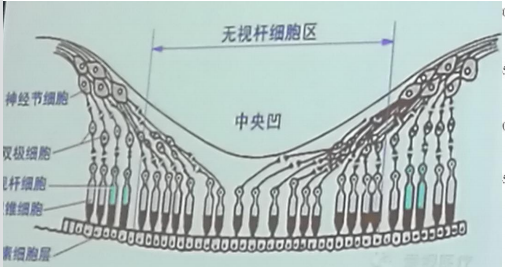graph LR
A[光信号] --> B[晶状体透镜]
B --> C[中间凹区域]
C --> D[光接收器]
D --> E[电脉冲信号]
E --> F[大脑解码]


### 视觉特性

• 亮度适应

人能感受到的光亮度最大值与最小值之比为$10^{10}$，随着光线亮度增加，人眼感受到的主观亮度对数增加。

$$主观亮度=K\cdot ln(客观亮度) + k_0$$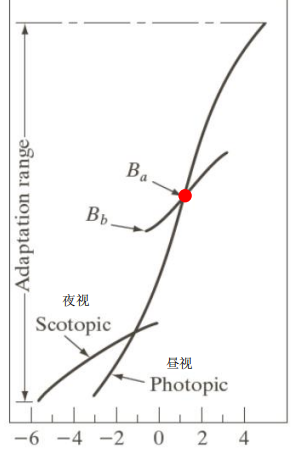• 马赫带现象：边界处，主观亮度对比强。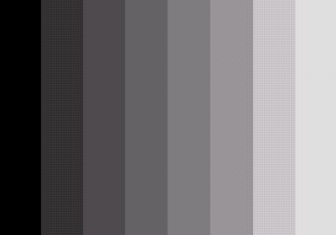• 同时对比现象：对于相同的亮度，背景变化时主观亮度会变化。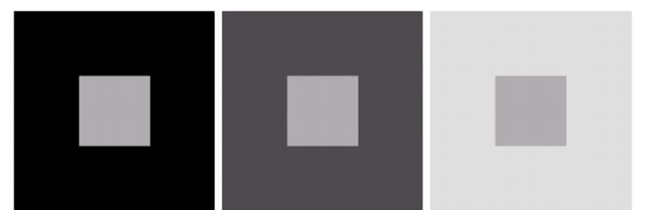### 图像内插

• 最近邻插值：选择最近邻点的灰度值
• 双线性插值：找到最近的4个点，进行加权平均，$v(x,y)=ax+by+cxy+d$
• 双三次内插：找到最近的16个点$\sum^3_i\sum^3_j a_{ij}x^iy^j$

### 像素空间基本关系

• 4邻域：$N_4(p)$上下左右
• 4对角邻域：$N_D{(p)}$左上 左下 右上 右下
• 8邻域：$N_8(p)$周围一圈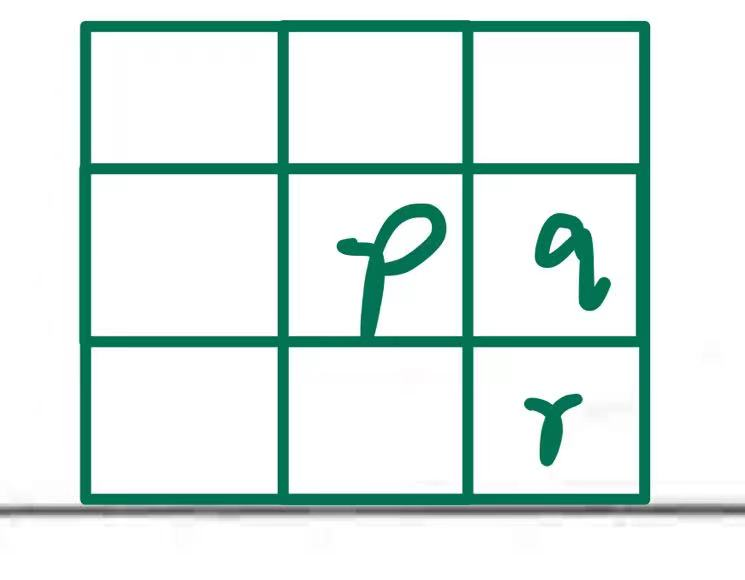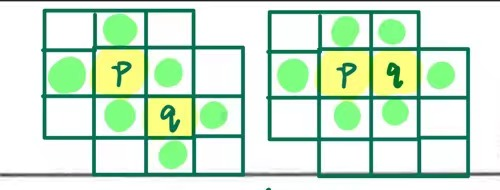• 4邻接：若p、q位置如图且灰度相似
• 8邻接：若p、r位置如图且灰度相似
• m邻接：若q在$N_4(p)$中或者q在$N_d(p)$中，且绿色部分（4邻域）没有灰度相似的像素。

1. 欧氏距离，圆形
2. $D_4$距离，$D_4(p,q)=|x-s|+|y-t|$，菱形，城市街区距离
3. $D_8$距离，$D_8(p,q)=max(|x-s|,|y-t|)$，方形，棋盘距离

## 第三章 灰度变换 空间滤波

$$g(x,y)=T[f(x,y)]$$### 灰度变换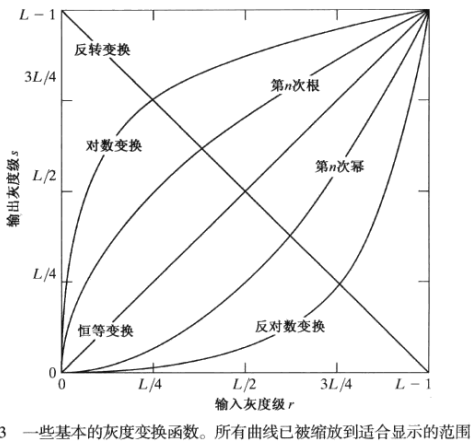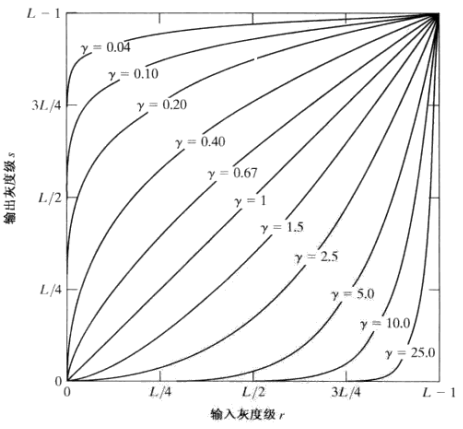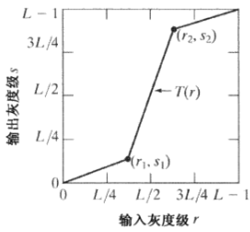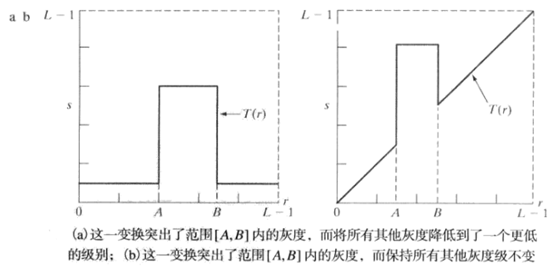#### 位平面分解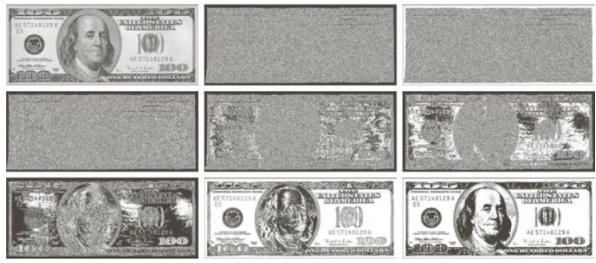#### 直方图均衡

1. 计算直方图$f(n_k)$
2. 计算灰度分布频率$p_r(k)=f(n_k)/N$。
3. 计算累计灰度分布频率$g_k=\sum p_r(k_i)$。
4. 计算均衡后图像直方图$round(g_k\cdot (L-1))$。L为灰度层数。四舍五入累计概率×灰度最大值。
5. 根据计算出的均衡直方图，将原图灰度映射到新图

#### 直方图统计

$$g(x,y)=\begin{cases}E\cdot f(x,y), \quad m \le k_0m_G\ and\ k_1\sigma_G \le \sigma \le k_2\sigma_G \ f(x,y),\qquad otherwise\end{cases}$$

### 空间滤波

$\begin{matrix} 1&1&1\1&-8&1\1&1&1\ \end{matrix}$ 加对角线的版本

Sobel锐化 $\begin{matrix} -1&-2&-1\0&0&0\1&2&1\ \end{matrix}$ x方向的一阶微分（需要加原图像
$\begin{matrix} -1&0&1\-2&0&2\-1&0&1\ \end{matrix}$ y方向的版本
$\begin{matrix} -2&-1&0\-1&0&1\0&1&2\ \end{matrix}$ 斜着的版本，朝着另一个方向斜也可以

（注意下面和右边是正数）

#### 非锐化屏蔽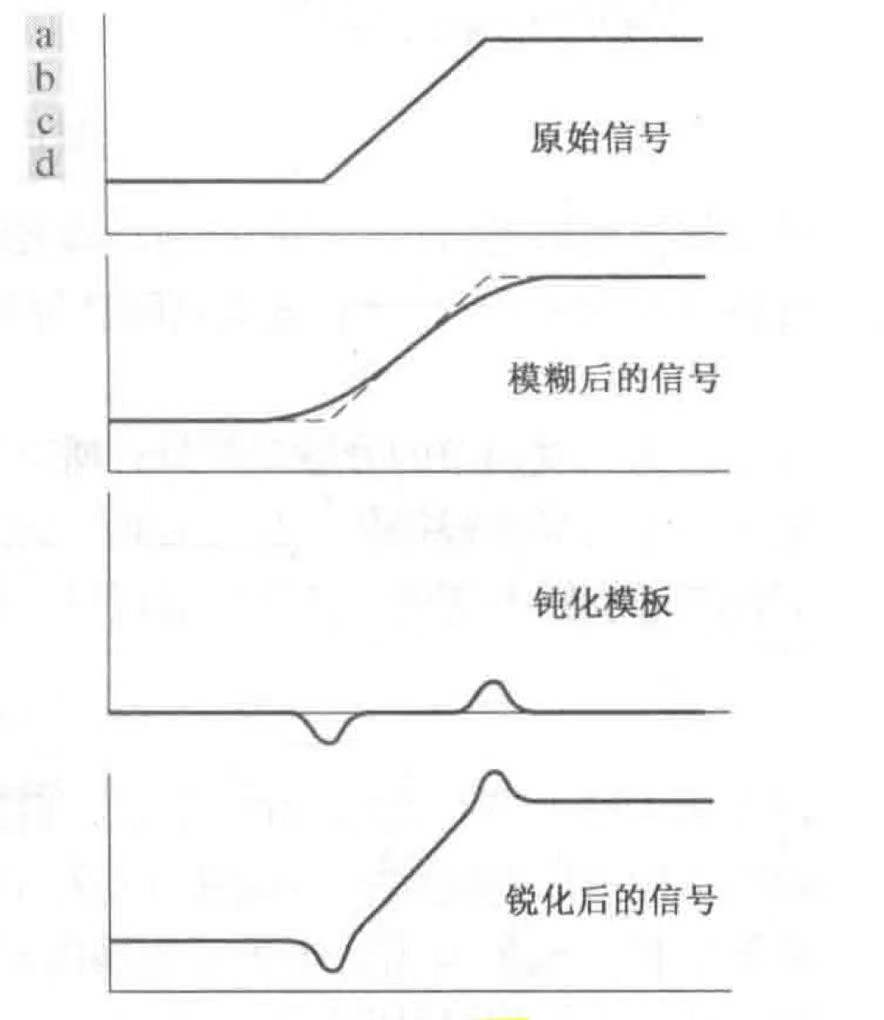## 第四章 频率域滤波

$$\bold{IDFT}(H(u,v) \cdot \bold{DFT}(f(x,y)))$$

### 高频强调滤波器

$$g(x,y)=\bold{IDFT}{[k_1+k_2H_{hp}(u,v)]F(u,v)}$$

$k_2$控制高频贡献，$k_1$控制原点偏移量，使零频率不被过滤。

### 同态滤波

“同态”指的是特殊的映射，比如$f(x)=e^x$，有$f(a+b)=f(a)\times f(b)$，把原本的加法映射成乘法。

1. 图像建模为$f(x,y)=i_{照射}(x,y)r_{反射}(x,y)$，使用对数操作转换成相加$ln\ f(x,y)=ln\ i(x,y)+ln\ r(x,y)$，这一步就是“同态”的关键。

2. 然后就可以用傅里叶变换了$\bold{FT}[ln\ f(x,y)]=\bold{FT}[ln\ i(x,y)]+\bold{FT}[ln\ r(x,y)]$。把上式记作$Z(u,v)=F_i(u,v)+F_r(u,v)$，则用可以用滤波器进行处理$H(u,v)Z(u,v)=H(u,v)F_i(u,v)+H(u,v)F_r(u,v)$。

3. 对于$F_i(u,v)$和$F_r(u,v)$，低频成分通常与照射光有关，而高频成分与反射相联系，所以使用修改过后的高斯高通滤波器作为$H(u,v)$。之后就傅里叶反变换回去$\bold{IFT}[H\cdot Z]=\bold{IFT}[H\cdot F_i]+\bold{IFT}[H\cdot F_r]$。再通过取指数把加法转换回乘法。

## 第五章 图像复原与重建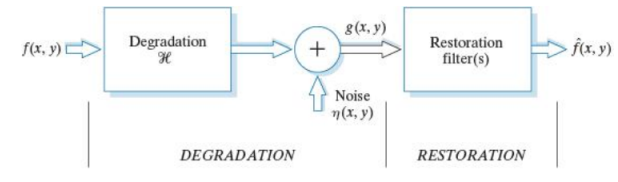$g(x,y)$是退化图像。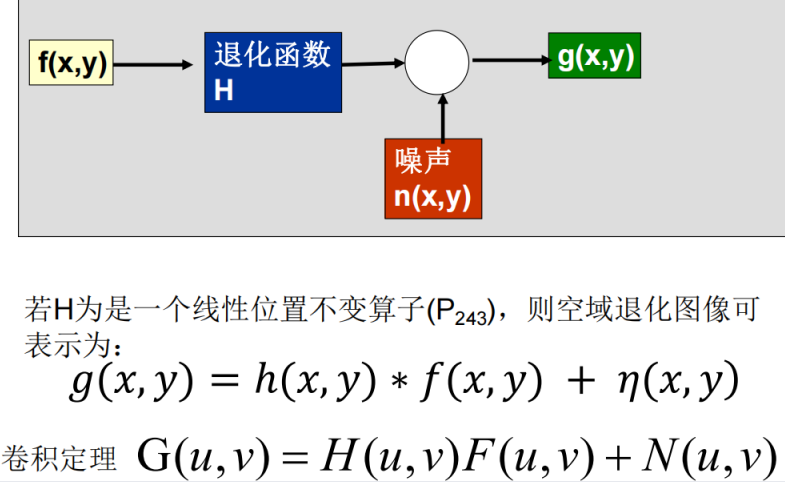### 自适应中值滤波器

1. 选择窗口$S_{xy}$，找到中值$z_{med}$，假如中值不符合$z_{min}<z_{med}<z_{max}$，则扩大窗口再选中值，直到窗口大小到达规定的$S_{max}$。
2. 找到中值之后，判断窗口中心点符不符合$z_{min}<z_{xy}<z_{max}$，假如符合就不变，不符合就改成之前找到的中值。

### 维纳滤波

$$\hat F(u,v)=\frac{|H|^2}{|H|^2+\frac{S_\eta}{S_f}}\cdot \frac{G(u,v)}{H}$$

### 求二维傅里叶幅度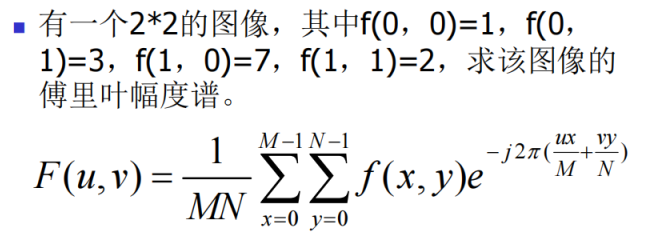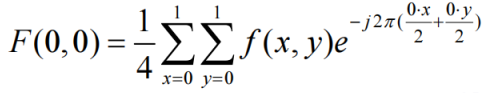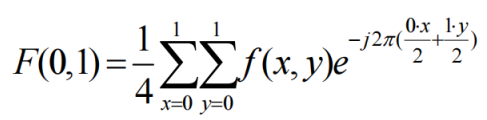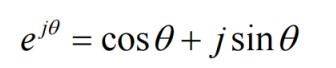## 第六章 彩色图像处理

### 彩色模型

#### CMY(K)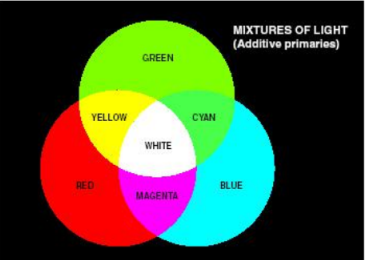#### YCbCr

JPEG使用的彩色空间，Y和YUV一样，而Cb是U乘一个常数，Cr是V乘一个常数。

### 全彩色图像处理

1. 可以分别处理每一个分量
2. 也可以统一处理是三个分量
3. 也可以只处理一个分量（比如调整亮度分量，做直方图均衡；或者把饱和度分量提高，增强对比度）

## 第九章 形态学滤波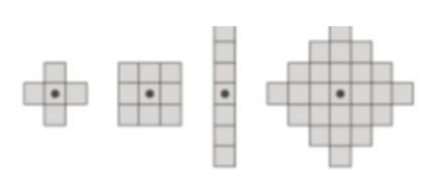### 逻辑运算

#### 击中hit、击不中miss、包含fit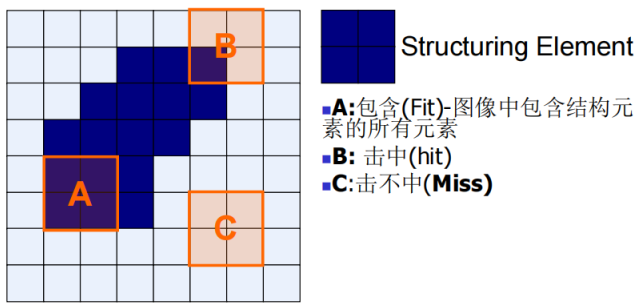#### 开运算、闭运算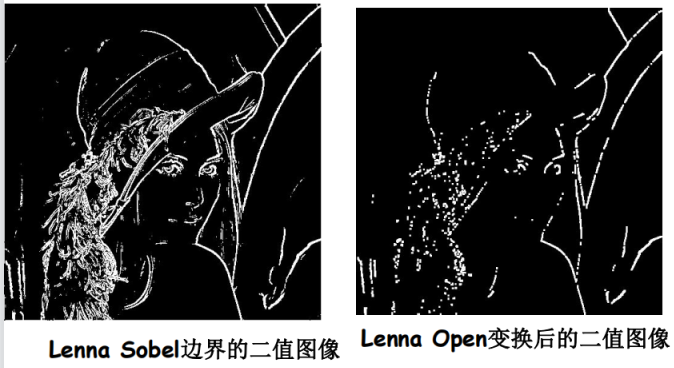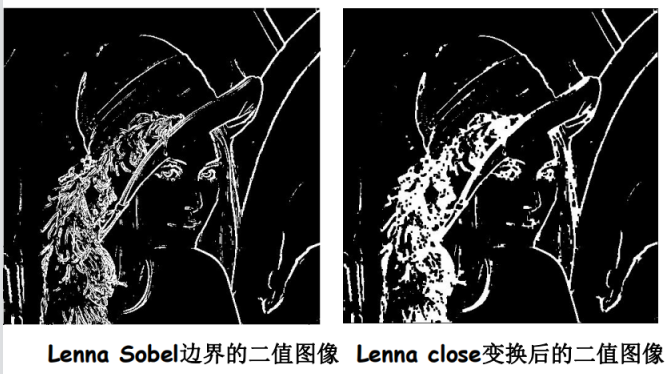### 应用

#### 边界提取

$$A-(A\ominus se)$$

#### 孔洞填充

1. 先选择一个在孔洞内的点集合$X_{0}$。
2. $X_k=(X_{k-1}\oplus se) \cap \overline A$。
3. 假如$X_k=X_{k-1}$，算法结束，取$X_k \cup A$作为结果。

#### 提取连通分量

1. 先选择一个在所需连通分量里的点集合$X_0$
2. $X_k=(X_{k-1}\oplus se) \cap A$。
3. 假如$X_k=X_{k-1}$，算法结束，取$X_k$作为结果。

## 第十章 图像分割

### 点的检测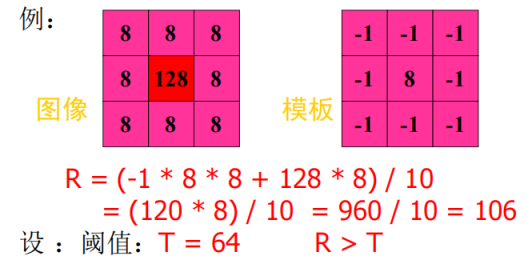### 线的检测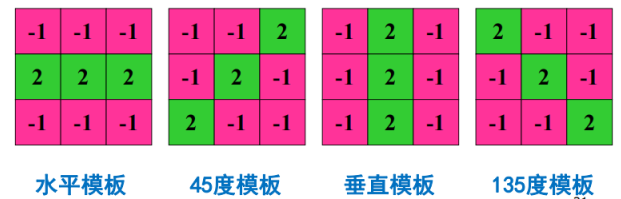### 边缘检测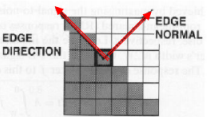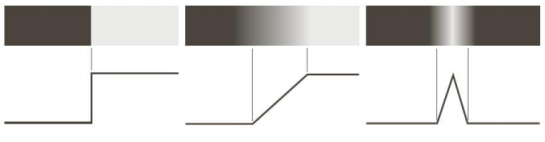• 平滑降噪：基于导数计算，所以容易受到噪声影响，但是平滑的同时也导致边缘强度的损失。
• 边缘点检测：微分算子能够突出边缘，然后通过设置阈值来提取边缘点。
• 边缘定位：精确确定边缘的位置。从候选点集中选择真实成员。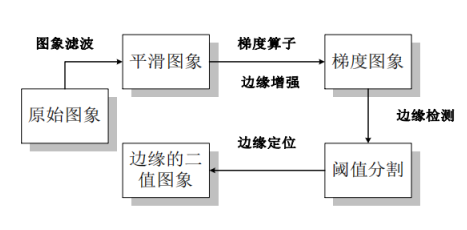#### Sobel梯度法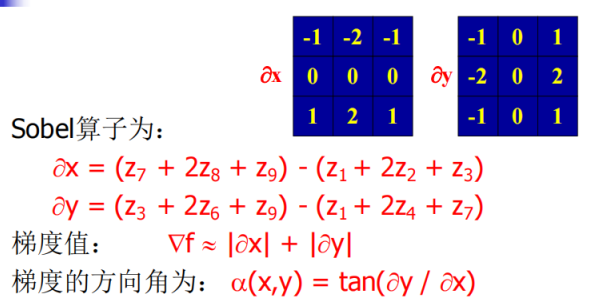Sobel既能提取x和y方向的微分，还有一定的平滑效果。提取出梯度之后，设置一个阈值，大于threshold的就设置为1，其余为0.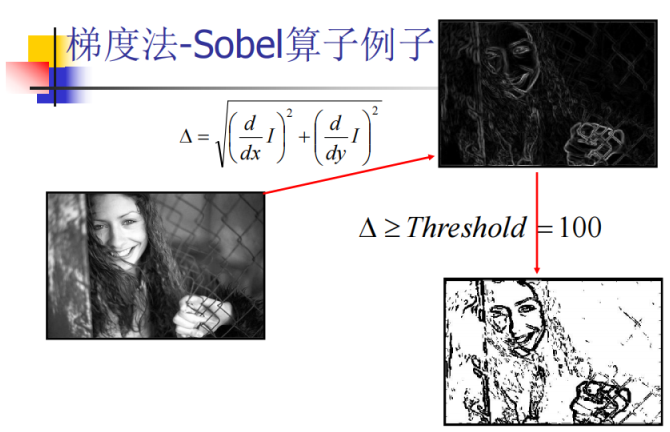#### 二阶导数 LoG法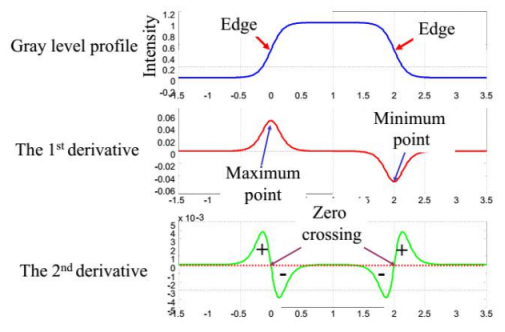1. 用高斯滤波器进行平滑降噪
2. 用二阶拉普拉斯检测边缘点
3. 根据二阶导数零交叉点定位边缘

$$G_\sigma(x,y)=\frac{1}{2\pi\sigma^2}\cdot exp(-\frac{x^2+y^2}{2\sigma^2}) \ \nabla^2G_\sigma(x,y)=\frac{1}{2\pi\sigma^4}(\frac{x^2+y^2}{\sigma^2}-2)\cdot exp(-\frac{x^2+y^2}{2\sigma^2})$$

#### Canny法

1. 用高斯滤波器进行平滑降噪
2. 用一阶偏导的有限差分计算梯度幅值和方向
3. 对梯度幅值进行非极大值抑制
4. 双阈值算法和连接分析来检测并连接边缘

#### 直方图阈值分割法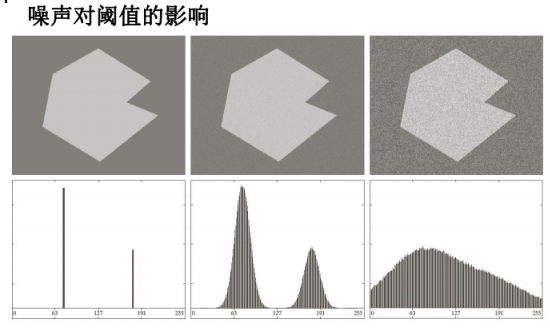#### Isodata门限算法

1. 选取门限的初值$T_0$
2. 用$T_i$分隔图像，分成$G_1$和$G_2$两类像素
3. 计算$G_1$和$G_2$的平均灰度$\mu_1$和$\mu_2$
4. 令新门限$T_{i+1}=\frac{1}{2}(\mu_1+\mu_2)$
5. 重复2-4，直至$\Delta T=0$

#### Otsu最佳全局阈值法

1. 计算输入图像的归一化直方图

2. 对于阈值$k=0,1,…,L-1$计算像素被分类到$[0,k]$的概率$P_1(k)$

3. 对于阈值$k=0,1,…,L-1$计算像素被分类到$[0,k]$的平均灰度$m(k)=\sum^{k}_{i=0}ip_i$

4. 计算整个图像的平均灰度$m_G=m(L-1)$

5. 对于阈值$k=0,1,…,L-1$计算类间方差
$$\sigma^2_B(k)=\frac{[m_GP_1(k)-m(k)]^2}{P_1(k)[1-P_1(k)]}$$
其实类间方差是由$\sigma_B^2=P_1(m_1-m_G)^2+P_2(m_2-m_G)^2$的化简得来的。

6. 让$\sigma_B^2(k)$最大的$k^*$值就是$Otsu$阈值。如果不唯一，则取每个$k^*$的平均。

#### 可变阈值处理